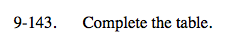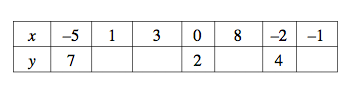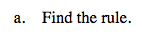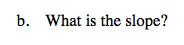### Home > CC3 > Chapter 9 > Lesson 9.2.6 > Problem9-143

9-143.
1.  x –5 1 3 0 8 –2 –1 y 7 2 4

Complete the table. 9-143 HW eTool (Desmos). Homework Help ✎

1. Find the rule.

2. What is the slope?Do you see any patterns?
Notice the difference in the y-value from x = 0 to x = −2.

Also notice that as the x-value decreases, the y-value increases, and vice versa.

y = −x + 2$\text{Slope = \frac{rise}{run}}$

−1

Complete the table in the eTool below.
Click the link at right for the full version of the eTool: CC3 9-143 HW eTool